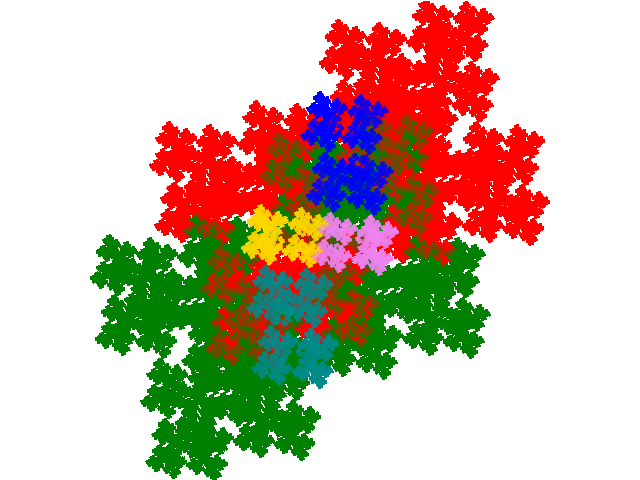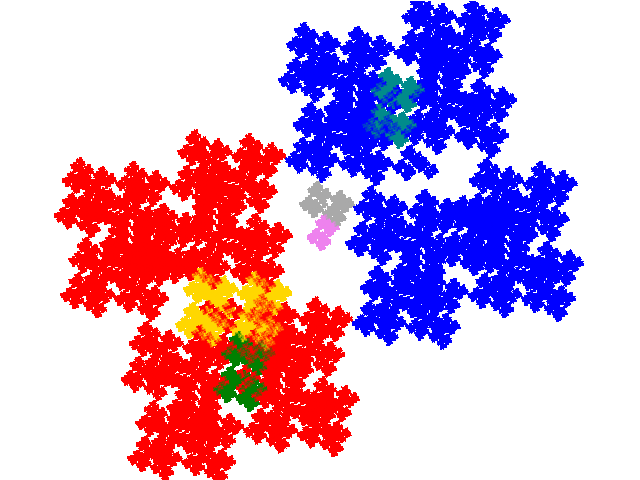# Some tiles associated with the 6th unit cubic Pisot number

## Allocomplex Tiles

There are 3 complex teragons among the order 3 sixth cubic tiles, one of which is the windowed tile. As a normal order 3 tile has 9 potential legitimate order 7 partial post(allo)composition derivatives, the remaining two have a potential 18 order 7 allodemisymmetric tiles. However 7 of the attractors are disconnected reducing the number to 11.

As the base tiles are complex teragons, all the derived tiles are complex teragons.

For the first complex tile there are 4 derived tiles. Two have the dissection equation c + 2c2 + c3 + 2c5 + c7 = 1 and tiling signature 001144.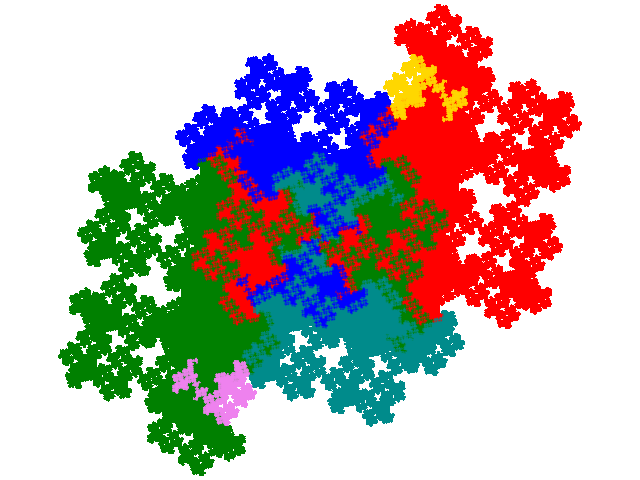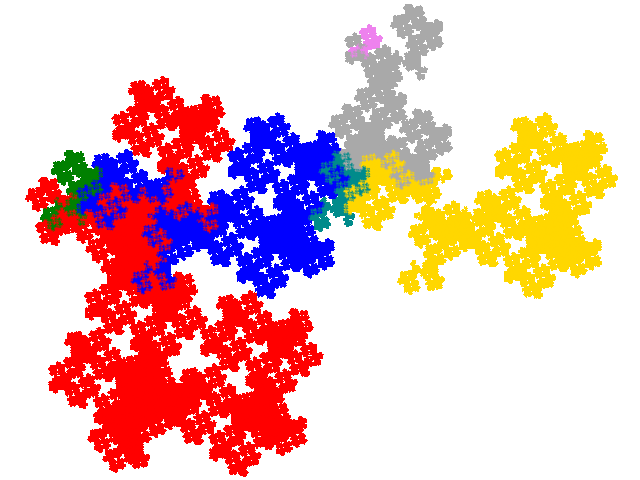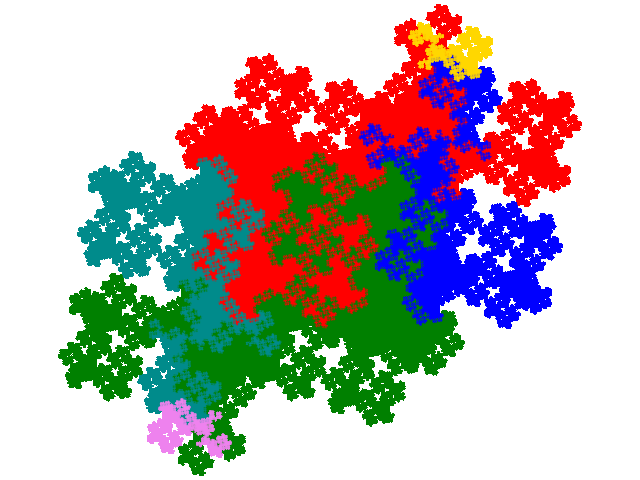A third has the dissection equation c + c2 + 3c3 + c4 + c5 = 1 and tiling signature 001122.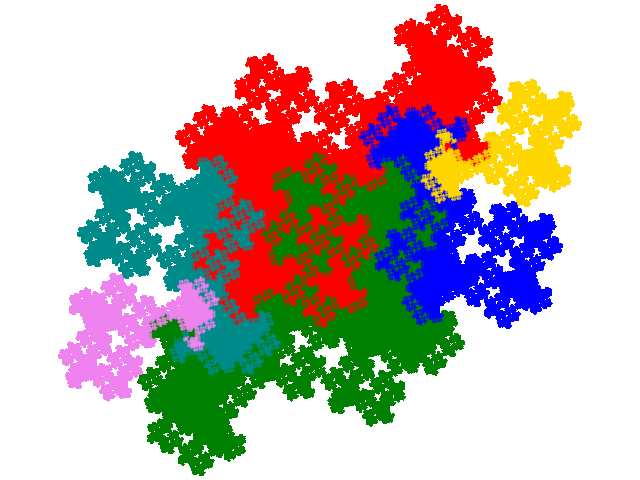The fourth has the dissection equation 2c + c4 + 2c5 + c6 + c7, and tiling signature 003344.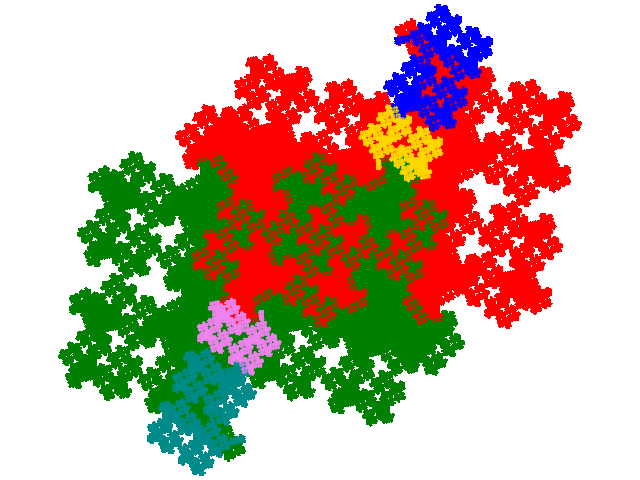As this first complex teragon has two alternative unit cells these four derived tiles also have alternative unit cells.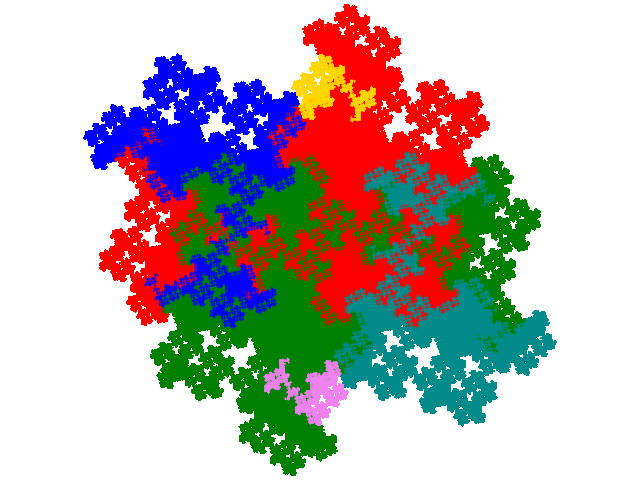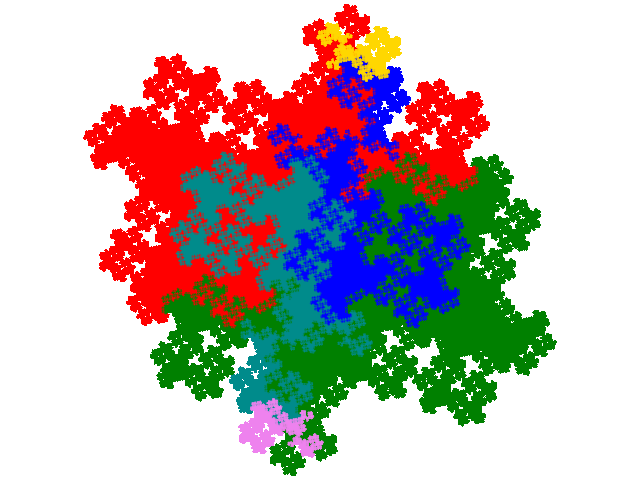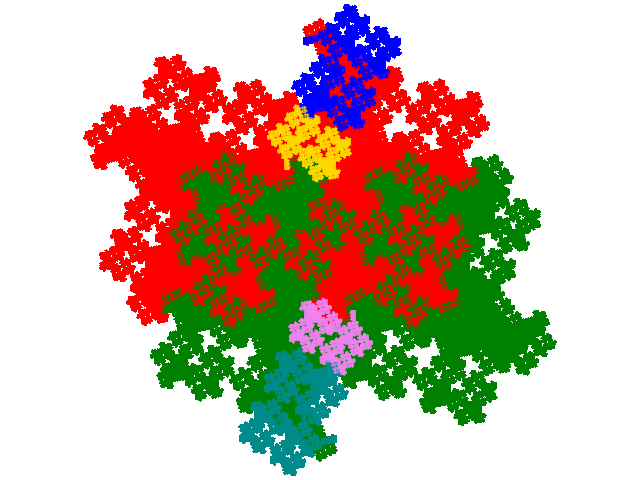The second complex teragon has 8 derived tiles. Four have have dissection equation c + c2 + 3c3 + c4 + c5 = 1 and tiling signature 001122.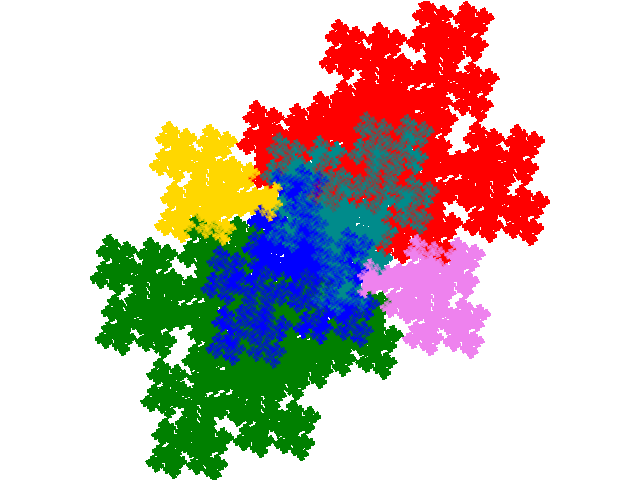Two have dissection equation c + 2c2 + c3 + 2c5 + c7, and tiling signature 001144.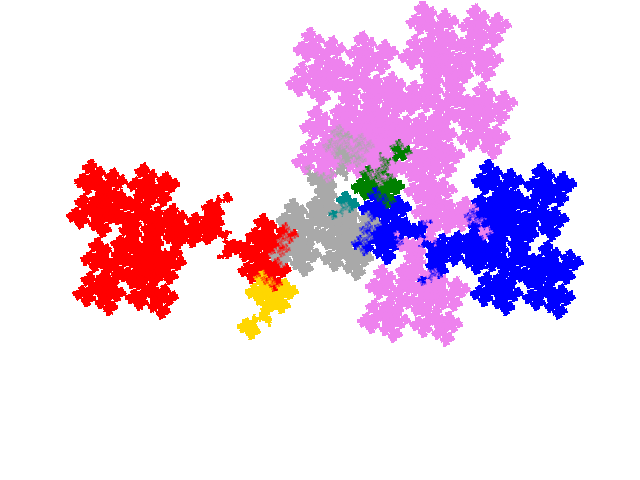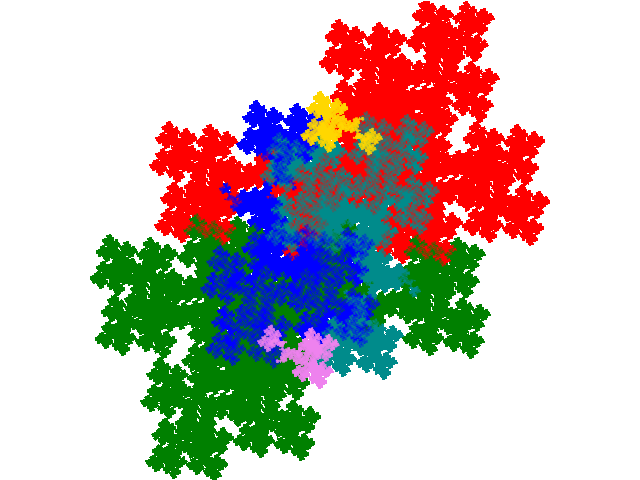and two have dissection equation 2c + c4 + 2c5 + c6 + c7, and tiling signature 003344.